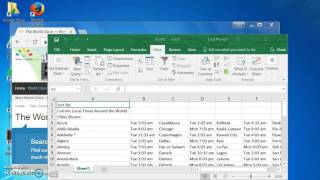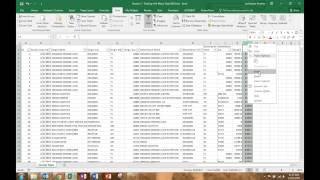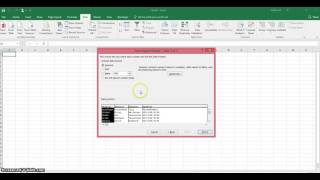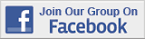Excel Tips & Tutorials:
 Suggest a Link Alphabetize Page Printer-Friendly List
Watch and Learn
Watch and Learn
Watch and Learn
Watch and Learn
 Importing Data from a Website to SpreadsheetLucy Provost demonstrates Excel 2016 web queries (6:38)Import Text Files into ExcelAjay Anand shows 5 different methods (13:07).Cleaning Messy Data for AnalysisAndrew Lockwood shares a lot of great tips and suggestions (21:10).How To Import A Text FileKeith Aul shows how to import data from plain text file (9:14).Importing Data from a Website to SpreadsheetLucy Provost demonstrates Excel 2016 web queries (6:38)Import Text Files into ExcelAjay Anand shows 5 different methods (13:07).Cleaning Messy Data for AnalysisAndrew Lockwood shares a lot of great tips and suggestions (21:10).Importing Data from a Website to SpreadsheetLucy Provost demonstrates Excel 2016 web queries (6:38)Import Text Files into ExcelAjay Anand shows 5 different methods (13:07).Importing Data from a Website to SpreadsheetLucy Provost demonstrates Excel 2016 web queries (6:38)RegressIt: Excel Add-On for Data Analysis Performs multivariate descriptive data analysis and regression analysis with high-quality table and chart output in...SolverStat SolverStat is an Excel add-in (xla) that performs advanced statistical tests on least squares regression data.RegressIt: Excel Add-On for Data Analysis Performs multivariate descriptive data analysis and regression analysis with high-quality table and chart output in...SolverStat SolverStat is an Excel add-in (xla) that performs advanced statistical tests on least squares regression data.RegressIt: Excel Add-On for Data Analysis Performs multivariate descriptive data analysis and regression analysis with high-quality table and chart output in...SolverStat SolverStat is an Excel add-in (xla) that performs advanced statistical tests on least squares regression data.RegressIt: Excel Add-On for Data Analysis Performs multivariate descriptive data analysis and regression analysis with high-quality table and chart output in...Over 18,000 Members!